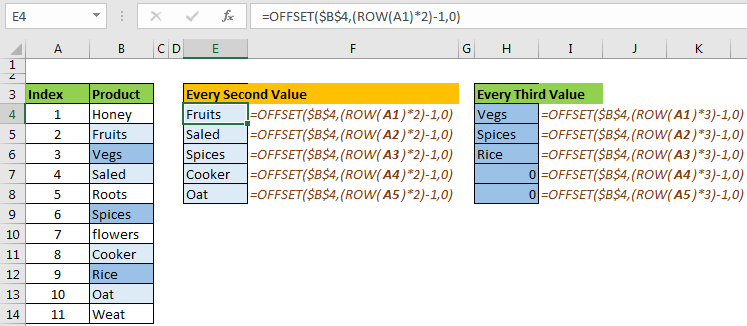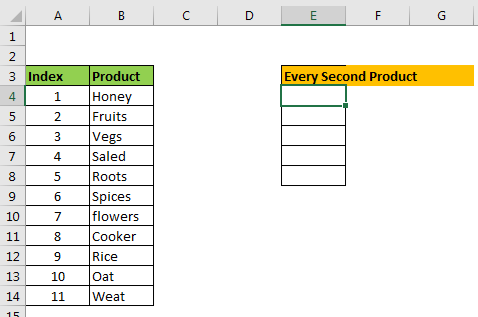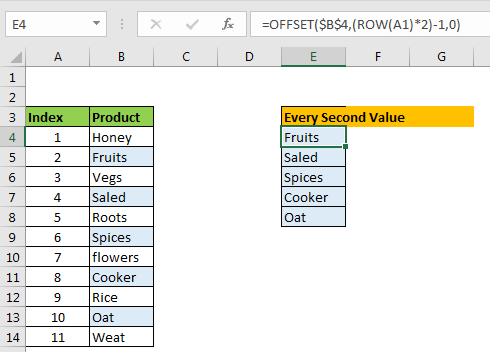# How to Get Every Nth Value From A listSome times we want to copy alternating cells from a range or every third value or every 4th value, and so on. In all these cases, copying manually will be a draining task. So what is the solution? How do you copy the value from every nth row? Is there a formula for this?

Yes, there is. We can use excel’s OFFSET function to get values from nth rows. How? Let’s see it.

=OFFSET(First_Cell_in_List,(ROW(A1)*N)-1,0)

First_Cell_in_Range: It is the absolute reference of the first cell in the list range from which you want to copy. For example, if your list is in A5:A100, then First_Cell_in_Range is \$A\$5. Do not include column heading.

N: it is of number which you’ll get multiples value. For example, if you want to get every 2nd value from the list, then N is 2. For every 3rd value, it is 3 and so on.

ROW(A1): it for getting multiples of N.

Now let’s see an example, and then I will explain how it works.

Example Copy Every Second Value from a List in Excel
Here we have a list of items. We need to copy every second value from the list in a new range.
So, how do we do that?Since we have 11 items on the list, we will get five alternate items (2, 4, 5, 6, 10).

Write the below formula in E4 and copy down the cells.

=OFFSET(\$B\$4,(ROW(A1)*2)-1,0)

Here \$B\$4 is the first cell in list range, and since we want to copy every second (alternate) value from the list, we have our N as 2.If you want to copy every third item, use this formula.

=OFFSET(\$B\$4,(ROW(A1)*3)-1,0)

For every 4th:

=OFFSET(\$B\$4,(ROW(A1)*4)-1,0)

And so on. You can copy any Nth Value from a using this excel formula.

How It Works

The formula accomplishes this task using two excel functions, OFFSET and ROW.

The OFFSET function returns the value from row and column offset from a given cell.

The ROW function returns the row number of given reference.

Starting from inside, ROW(A1) will return 1. Since A1 belongs to 1st row.

(ROW(A1)*2 it will become 2.

(ROW(A1)*2)-1, it will become (1*2)-1=1.

When we copy down the formula, the reference in ROW will change as A2, A3, A4, and so on. For A2, we will get the final value (2*2)-1=3. For A3, we will get (3*3)-1=5 and so on.

Now, our formula in E4 us solved to =OFFSET(\$B\$4,1,0). This will return the value 1 row below from B4, which is the second value from B4.

In E4, the formula will be  =OFFSET(\$B\$4,3,0), which is the fourth value from B4. Similarly, we get all the alternating costs.

Now you know how to copy every Nth value from the list in excel. This is the technique I know. If you know any other trick for getting copying nth cell from a range, feel free to share in the comments section below. If you have any queries regarding this or any other function of Excel 2019, 2016, 2013, and 2010, use the comments section.

Related Articles:

How to Retrieve Latest Price in Excel

Retrieving the First Value in a List that is Greater / Smaller than a Specified Value

How to retrieve the entire row of a matched value

Popular Articles:

The VLOOKUP Function in Excel

COUNTIF in Excel 2016

How to Use SUMIF Function in Excel

Terms and Conditions of use

The applications/code on this site are distributed as is and without warranties or liability. In no event shall the owner of the copyrights, or the authors of the applications/code be liable for any loss of profit, any problems or any damage resulting from the use or evaluation of the applications/code.